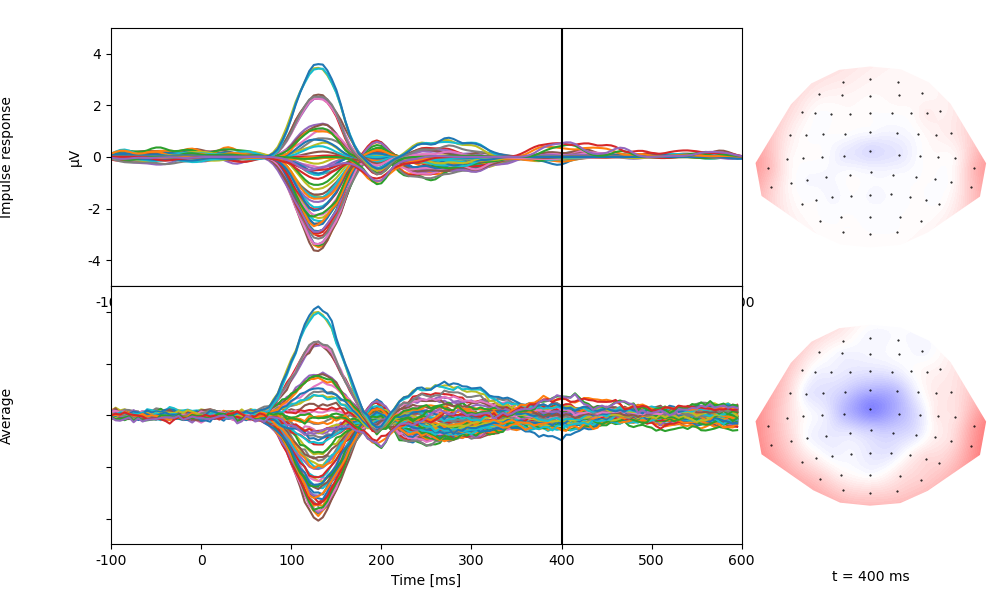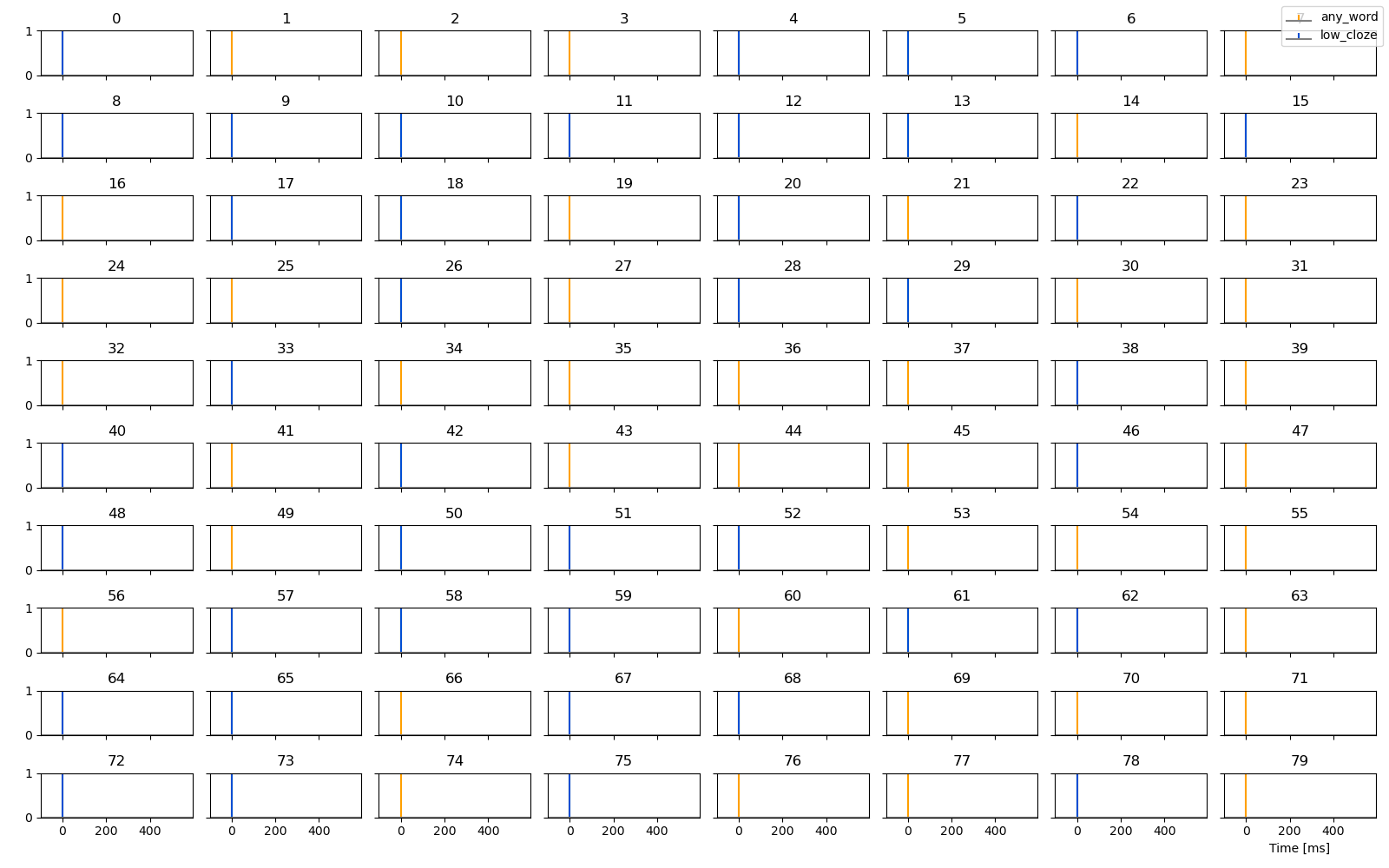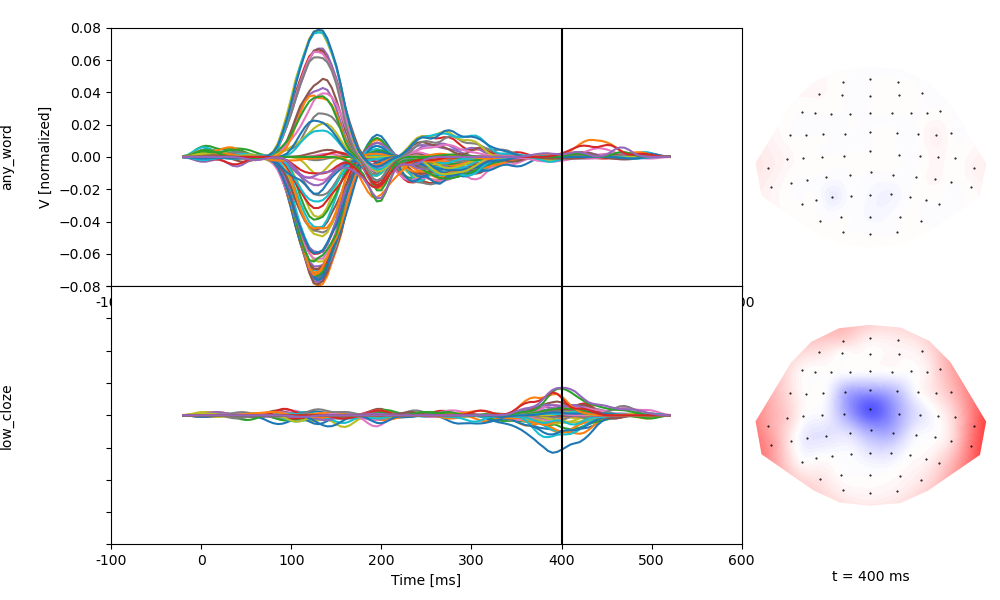# Impulse predictors for epochs

`epoch_impulse_predictor()` generates predictor variables for reverse correlation in trial-based experiments with discrete events. The function generates one impulse per trial, and these impulsescan be of varaiable magnitude and have variable latency.

The example uses simulated data meant to vaguely resemble data from an N400 experiment, but not intended as a physiologically realistic simulation.

```# sphinx_gallery_thumbnail_number = 2
from eelbrain import *

ds = datasets.simulate_erp()
print(ds.summary())
```
```Key         Type     Values
--------------------------------------------------------------------
eeg         NDVar    140 time, 65 sensor; -1.85296e-05 - 2.11533e-05
cloze       Var      0.00563694 - 0.997675
cloze_cat   Factor   high:40, low:40
n_chars     Var      0:2, 1:5, 2:6, 3:13, 4:23, 5:20, 6:7, 7:4
--------------------------------------------------------------------
Dataset: 80 cases
```

## Discrete events

Computing a TRF for an impulse at trial onset is very similar to averaging:

```any_trial = epoch_impulse_predictor('eeg', 1, ds=ds)
fit = boosting('eeg', any_trial, -0.100, 0.600, basis=0.050, ds=ds, partitions=2, delta=0.01)
average = ds['eeg'].mean('case')
trf = fit.h.sub(time=(average.time.tmin, average.time.tstop))
p = plot.TopoButterfly([fit.h_scaled, average], xlim=(-0.100, 0.600), axtitle=['Impulse response', 'Average'], t=0.400)
```## Categorial coding

Impulse predictors can be used like dummy codes in a regression model. Use one impulse to code for occurrence of any word (`any_word`), and a second impulse to code for unpredictable words only (`cloze`):

```any_word = epoch_impulse_predictor('eeg', 1, ds=ds, name='any_word')
# effect code for cloze (1 for low cloze, -1 for high cloze)
cloze_code = Var.from_dict(ds['cloze_cat'], {'high': 0, 'low': 1})
low_cloze = epoch_impulse_predictor('eeg', cloze_code, ds=ds, name='low_cloze')

# plot the predictors for each trial
plot.UTS([any_word, low_cloze], '.case')
``````<UTS: any_word, low_cloze ~ .case>
```

Estimate response functions for these two predictors. Based on the coding, `any_word` reflects the response to predictable words, and `low_cloze` reflects how unpredictable words differ from predictable words:

```fit = boosting('eeg', [any_word, low_cloze], 0, 0.5, basis=0.050, model='cloze_cat', ds=ds, partitions=2, delta=0.01)
p = plot.TopoButterfly(fit.h, xlim=(-0.100, 0.600), t=0.400)
```Total running time of the script: ( 0 minutes 10.662 seconds)

Gallery generated by Sphinx-Gallery